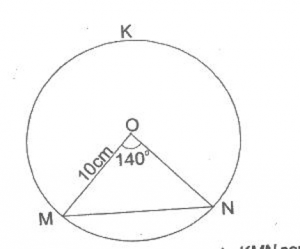# Mathematics 2017 Past Questions | JAMB

Study the following Mathematics past questions and answers for JAMBWAEC  NECO and Post-JAMB. Get prepared with official past questions and answers for upcoming examinations.

31. In how many ways can the word MATHEMATICS be arranged?

• A. $$\frac{11!}{9!2!}$$
• B. $$\frac{11!}{9!2!2!}$$
• C. $$\frac{11!}{2!2!2!}$$
• D. $$\frac{11!}{2!2!}$$

32.  In how many ways can the word MACICITA be arranged?

• A. $$\frac{8!}{2!}$$
• B. $$\frac{8!}{3! 2!}$$
• C. $$\frac{8!}{2! 2! 2!}$$
• D. 8!

33.  y is inversely proportional to x and y and 6 when x = 7. Find the constant of the variation

• A. 47
• B. 42
• C. 54
• D. 46

34.In the diagram MN, PQ and RS are parallel lines. What is the value of the angle marked X?
• A. 123o
• B. 170o
• C. 117o
• D. 137o

35. Find the equation of the locus of a point p (x, y) such that pv = pw, where v= (1, 1) and w = (3, 5)

• A. 2x + 2y = 9
• B. 2x + 3y = 8
• C. 2x + y = 9
• D. x + 2y = 8

36. Find ∫(x2 + 3x − 5)dx

• A. $$\frac{x_3}{3}$$ – $$\frac{3x_2}{2}$$ – 5x + k
• B. $$\frac{x_3}{3}$$ – $$\frac{3x_2}{2}$$ + 5x + k
• C. $$\frac{x_3}{3}$$ + $$\frac{3x_2}{2}$$ – 5x + k
• D. $$\frac{x_3}{3}$$ + $$\frac{3x_2}{2}$$ + 5x + k

37.In the diagram above MN is a chord of a circle KMN centre O and radius 10cm. If < MON = 140°, find, to the nearest cm, the length of the chord MN.

• A. 10cm
• B. 18cm
• C. 17cm
• D. 12cm

38. If m * n = [mn − nm] for m, n belong to R, evaluate − 3 * 4

• A. 3
• B. 4
• C. 5
• D. 6

39. Factorize completely x2 + 12xy + y2 + 3x + 3y – 18

• A. (x + y + 6)(x + y -3)
• B. (x – y – 6)(x – y + 3)
• C. (x – y + 6)(x – y – 3)
• D. (x + y – 6)(x + y + 3)

40. Make S the subject of the relation
p = s + $$\frac{sm^2}{nr}$$

• A. s = $$\frac{nrp}{nr + m^2}$$
• B. s = nr + $$\frac{m^2}{mrp}$$
• C. s = $$\frac{nrp}{mr}$$ + m2
• D. s = $$\frac{nrp}{nr}$$ + m2
Subscribe
Notify of